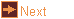# off_query - list element data

 Usage    |    Examples    |    Notes

## Usage

```

Usage: off_query [options] query [input_file]

Read a file in OFF format and list element data for specified elements.
Added elements of the query type are also added to the query list.
Added elements are referred to by xN, where N is the order the element
was added (starting with 0). Query is a list of letters, the first is the
query element type V, F, or E followed by the values to print
V - Vertex:
c - coordinates             d - distance from centre
f - face index numbs        F - face angles
a - solid angle             n - neighbours
o - order                   g - vertex figure
K - colour
E - Edge:
v - vertex index nums       d - distance from centre
f - face index nums         D - direction
a - diheral angle           l - length
c - central angle           C - centroid
K - colour
F - Face:
v - vertex index nums       d - distance from centre
n - neighbours              A - area
N - normal                  C - centroid
a - angles                  l - lengths
s - number of sides         p - max nonplanar distance
P - perimeter               K - colour

Options
-h,--help this help message (run 'off_util -H help' for general help)
--version version information
-c <cent> centre of shape in form 'X,Y,Z', 0 to use origin, C to use
centroid (default 0)
-I <idxs> list of elements by index number given as index ranges
separated by commas, range can be one number or two
numbers separated by a hyphen (default range numbers: 0 and
largest index, default argument: any added elements else '-')
-v <crds> add vertex, coordinates in form 'X,Y,Z'
-f <vnos> add face, a list of vertex index numbers separated by commas
-e <vnos> add edges, a list of vertex index numbers separated by commas
and taken in pairs
-k        keep orientation, don't try to orient the faces
-E <type> edges for query, e - explicit edges, i - implicit edges
a - explicit and implicit (default)
-o <file> write output to file (default: write to standard output)
-d <dgts> number of significant digits (default 17) or if negative
then the number of digits after the decimal point

```

## Examples

List the angles around each vertex of a cuboctahedron
```off_query -I - VF -d 6 cubo
```

List the edge lengths of edges 5 to 10, 50 to 60 and 100 of a geodesic sphere
```off_query -I 5-10,50-60,100 El -d 6 geo_4_1
```

Find the distance between opposite vertices of a unit-edged icosahedron.
```off_trans -s e icosahedron | off_query -e 0,11 El
```

Find the angles between three vertices not on the same face
```off_trans -s e icosahedron | off_query -f 0,1,11 Fa
```

Find the angles between two vertices and a supplied vertex
```off_trans -s e icosahedron | off_query -v 1.0,0.0,0.0 -f 0,E0,11 Fa
```

## Notes

Use with caution! This program hasn't had extensive testing. It is very possible that some calculations may fail under particular conditions. Please, report any bugs.